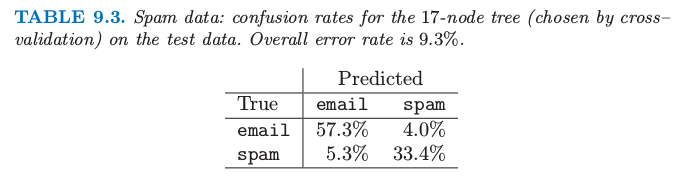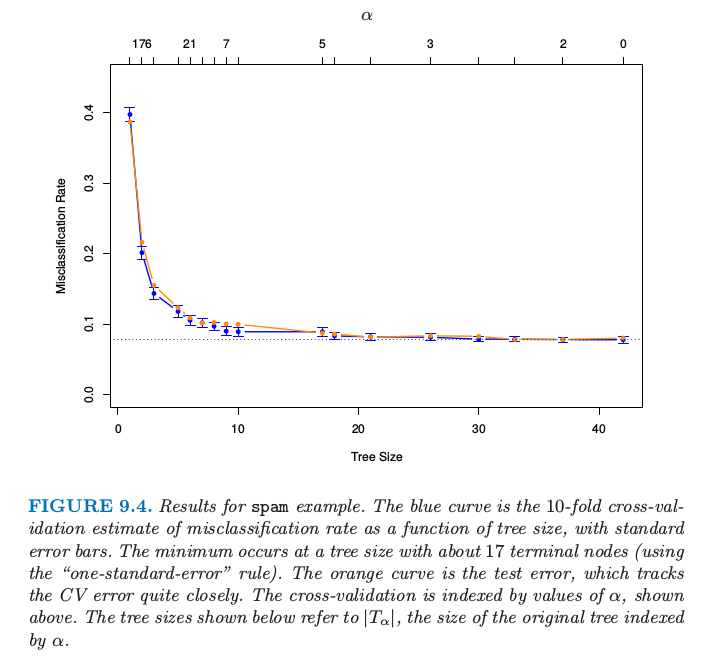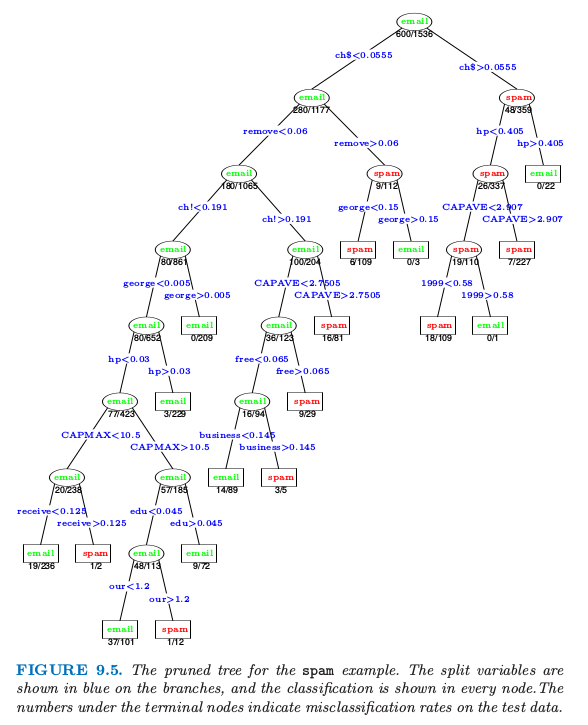# 9.2 基于树的方法(CART)¶

## 背景¶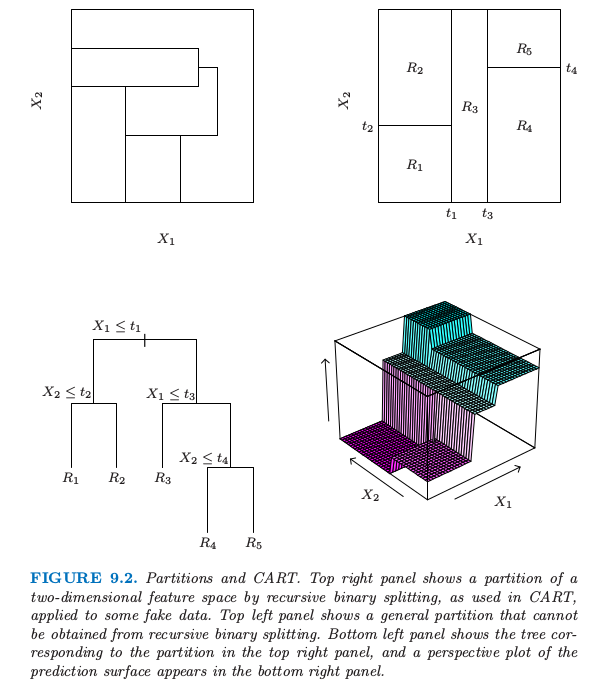## 分类树¶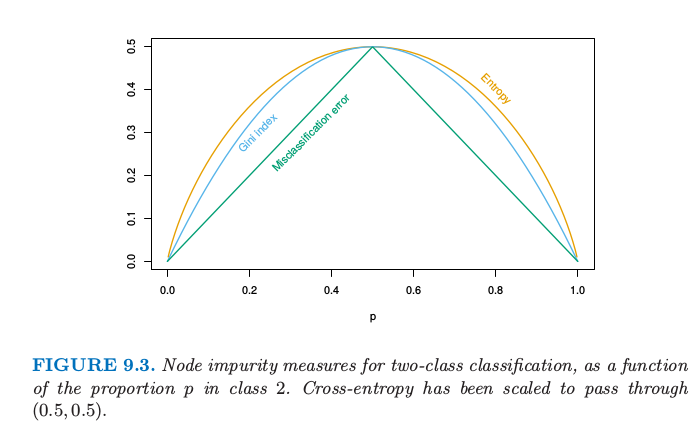Gini指数可以用两种方式来解读．与其将观测值划分到结点的主要类别中，我们可以以一定概率$\hat p_{mk}$将它们划分到类别$k$．于是该结点的这条规则的训练误差率是$\sum_{k\neq k’}\hat p_{mk}\hat p_{mk’}$——Gini指数．类似地，如果我们对类别$k$的观测编码为1，而对其它的观测编码为0，则该结点的0-1响应变量的方差为$\hat p_{mk}(1-\hat p_{mk})$.将$k$个类别的全部相加再一次得到Gini指数．

weiya注

library(rpart)

library(rpart.plot)

fit <- rpart(Kyphosis ~ Age + Number + Start, data = kyphosis)

rpart.plot(fit)

## 垃圾邮件的例子（继续）¶